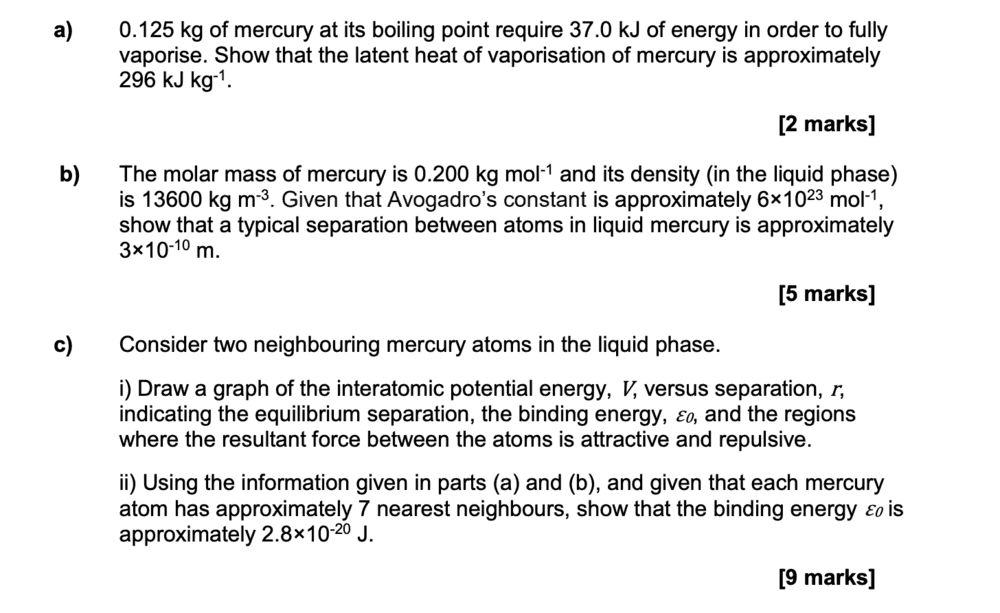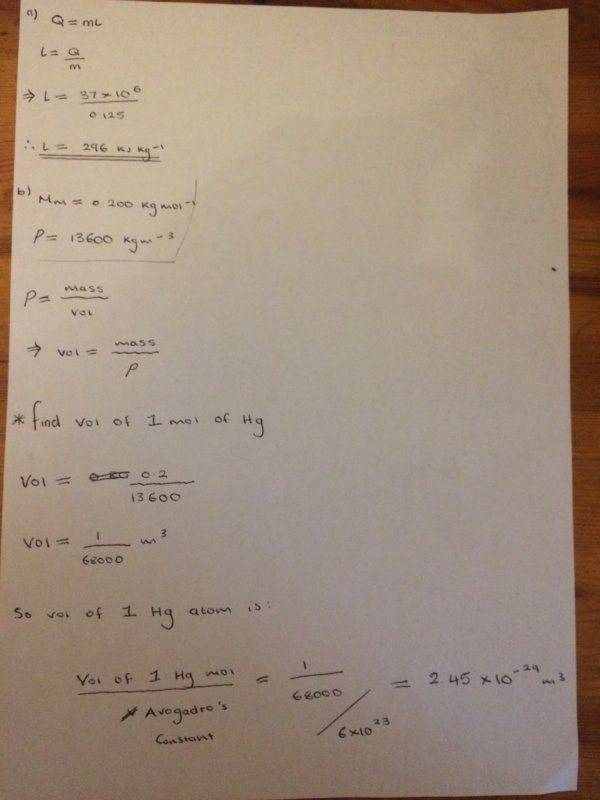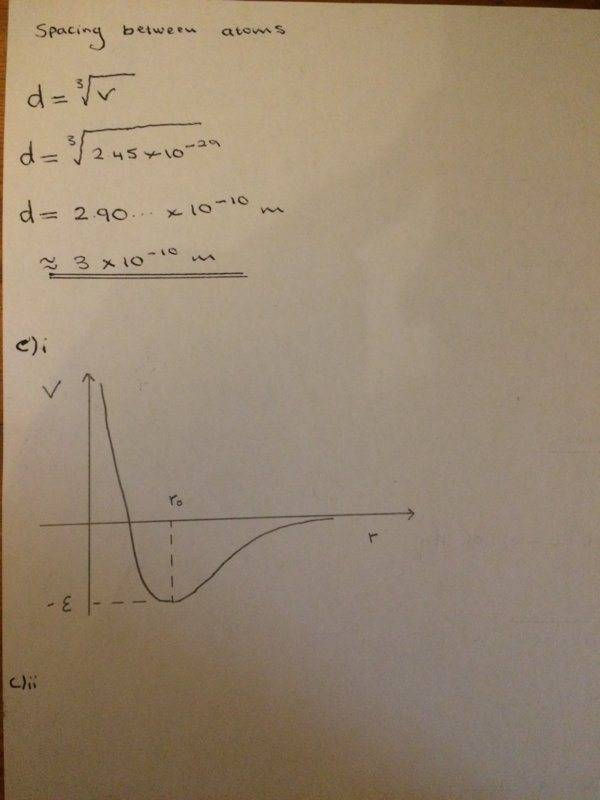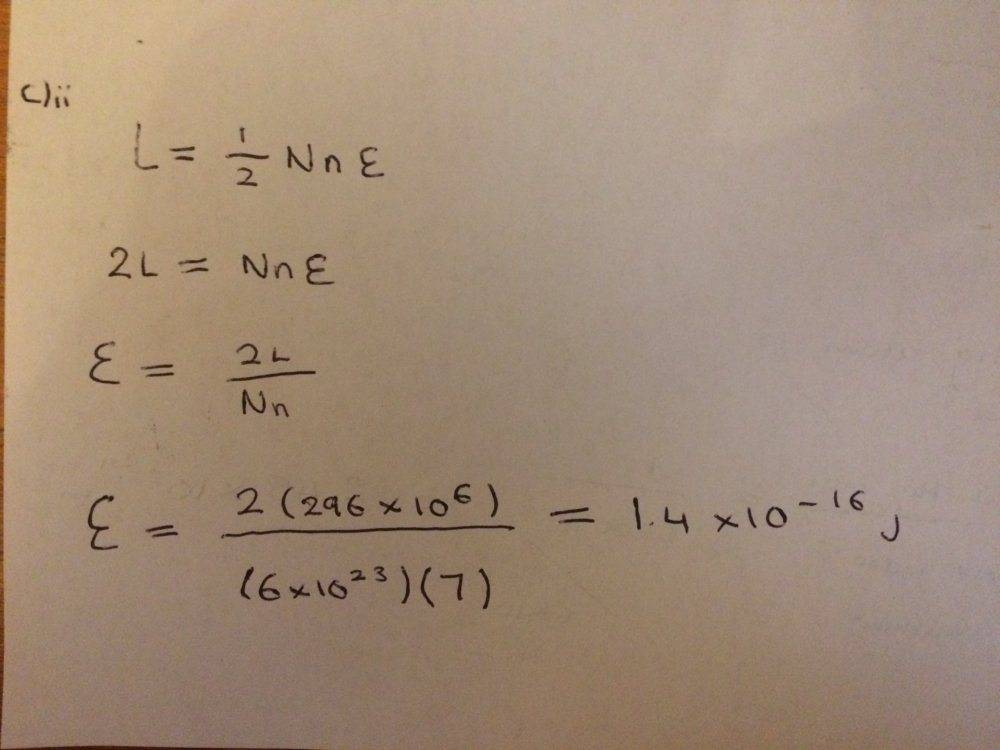Bolter
Homework Statement:
Working out how to calculate binding energy
Relevant Equations:
l = 1/2Nne
Here is the question:Stuck on how to complete part c)ii

Here is what I have done so far as well as trying to answer part c)iiAny help would be appreciated! Thanks

Homework Helper
Gold Member
37 kJ = 37x103 J.

•berkeman
Bolter
37 kJ = 37x103 J.

Oh shoot yes you’re right. I don’t know why I processed it at as mega joules

So doing the following calculation again gives

Binding energy = (2 * 293000) / (6x10^23 * 7) = 1.41x10^-19 J which is closer to the answer but still not right

Homework Helper
296 kJ/kg. You need kJ/mol.

Bolter
296 kJ/kg. You need kJ/mol.

So to convert from kJ/kg to kJ/mol, I must multiply kJ/kg by the molar mass?

I did this and got the following

Binding energy = 2(293000 * 0.20)/(6x10^23)(7) = 2.79... x10^-20 J which is roughly equal to 2.8x10^-20 J

I also got another part that I need help with if you don’t mind

Not entirely sure how to approach part d)ii but I suppose I had done part d) i right

#### Attachments

Homework Helper
di is right. Not sure what the problem is for dii - find the volume per atom, as you did for Hg.

Bolter
di is right. Not sure what the problem is for dii - find the volume per atom, as you did for Hg.

To find volume per atom. I know that 1.0691... x10^-4 moles of Hg is contained in 2x10^-4 m^3.

So to firstly get the volume per mole of Hg, I do ' 2x10^-4 / 1.0691...x10^-4 ' which gives 1.870... volume per mole

Dividing 1.870... by avogrado's constant is '1.870... / 6.022x10^23 = 3.106... x 10^-24 volume per atom
Cube rooting volume per atom number should give the spacing which comes out to be '1.459... x10^-8 m'

Would you agree that these steps are the right way of doing this?

Homework Helper
Yes, except that you're talking about neon, not mercury.

•Bolter
Bolter
Yes, except that you're talking about neon, not mercury.

Yes, sorry I got mixed up with the wording somehow

•